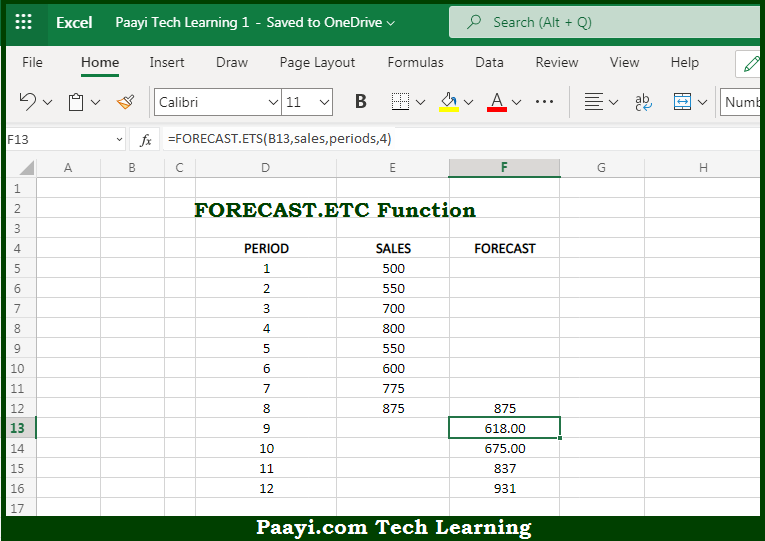# Learn How to Use Microsoft Excel FORECAST.ETS Function

Written by | 0 Comments | 620 Views

In this article, you will learn how to use Microsoft Excel FORECAST.ETS function and its prime function in Microsoft Excel. You will also get to know Microsoft Excel FORECAST.ETS function return value and syntax with the help of some examples.

Microsoft Excel FORECAST.ETS Function

The main purpose of the Microsoft Excel FORECAST.ETS function is to predict the value with a seasonal trend. That implies, with the help of FORECAST.ETS function you can able to predict a value based on already given values that follows the seasonal trend. The FORECAST.ETS function calculates future value predictions using a seasonal pattern and can be used to predict numeric values like sales, expenses, inventory, measurements, etc. So, with the help of FORECAST.ETS function, you can able to predict the value with a seasonal trend.

Return Value of FORECAST.ETS Function

The return value will be the predicted value.

Syntax of FORECAST.ETS Function

=FORECAST.ETS(target-date, values, timeline, [seasonality], [data-completion], [aggregation])

Where the arguments:

• target-date: This is the time or period for the prediction (x value).
• values: This is the existing or historical values (y values).
• timeline: This is the numeric timeline values (x values).
• seasonality: This is the Seasonality calculation, where 0 = no seasonality, 1 = automatic, n = season length in timeline units (optional).
• data-completion: This is the Missing data treatment, where, 0 = treat as zero, 1 = average and default is 1 (optional).
• aggregations: This is the Aggregation behavior. Default is 1 AVERAGE (optional).

## How to Use Microsoft Excel FORECAST.ETS Function?So we know that, Microsoft Excel FORECAST.ETS function you can able to predict the value with a seasonal trend. That implies, with the help of FORECAST.ETS function you can able to predict a value based on already given values that follows the seasonal trend. The FORECAST.ETS function calculates future value predictions using the seasonal patterns and can be used to predict numeric values like sales, expenses, inventory, measurements, etc. So, with the help of FORECAST.ETS function, you can able to predict the value with a seasonal trend.